For this hands-on exercise, we will use an adaptation of the pig_adg dataset described in Dohoo, Martin and Wayne - Veterinary Epidemiologic Research (second edition) see (Dohoo, Martin, Stryhn, & others, 2003).

Let’s start by loading the data into the working environment:

dt <- readRDS("Path_to_your_file/dataOK.RDS")

The data for this exercise consist of 341 observations of 12 variables. Here is an extract of the first rows:

head(dt)
# A tibble: 6 x 12
AR pneum pneumS female livdam  eggs  epg5 worms wormCount   age   adg
<dbl> <dbl>  <dbl>  <dbl>  <dbl> <dbl> <dbl> <dbl>     <dbl> <dbl> <dbl>
1     1     0      0      0      1     0     0     0         0   196   453
2     1     1      0      0      1     0     0     0         0   175   501
3     0     1      0      1      1     0     0     0         0   176   545
4     1     0      0      1      1     0     0     0         0   207   441
5     1     1      1      0      0     0     0     0         0   222   482
6     1     1      0      0      0     0     0     0         0   212   423
# ... with 1 more variable: farm <dbl>

The meaning of each variable is the following:

Variable Meaning
AR presence of atrophic rhinitis (0/1)
pneum presence of pneumonia (0/1)
pneumS presence of moderate to severe pneumonia (0/1)
female sex of the pig (1=female, 0=castrated)
livdam presence of liver damage (parasite-induced white spots) (0/1)
eggs presence of fecal/gastrointestinal nematode eggs at time of slaughter (0/1)
epg5 fecal gastriointestinal nematode egg count at time of slaughter (eggs/5g)
worms presece of nematodes in intestine (0/1)
wormCount count of nematodes in small intestine at time of slaughter (nr.)
age days elapsed from birth to slaughter (days)
adg average daily weight gain (grams)
farm farm ID

The distribution of each variable is the following: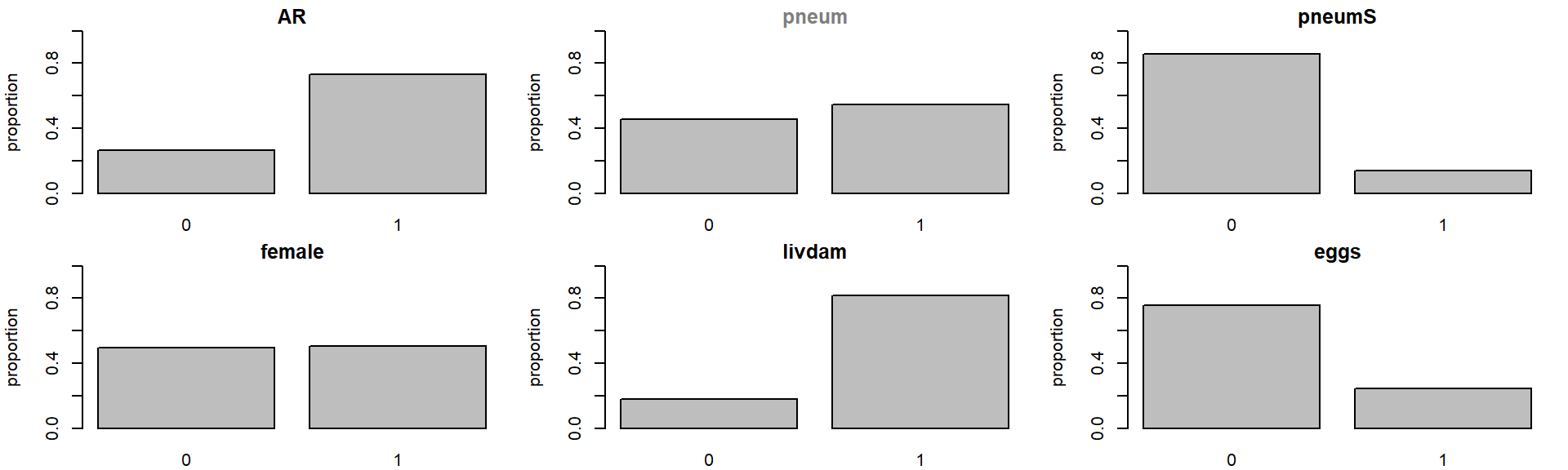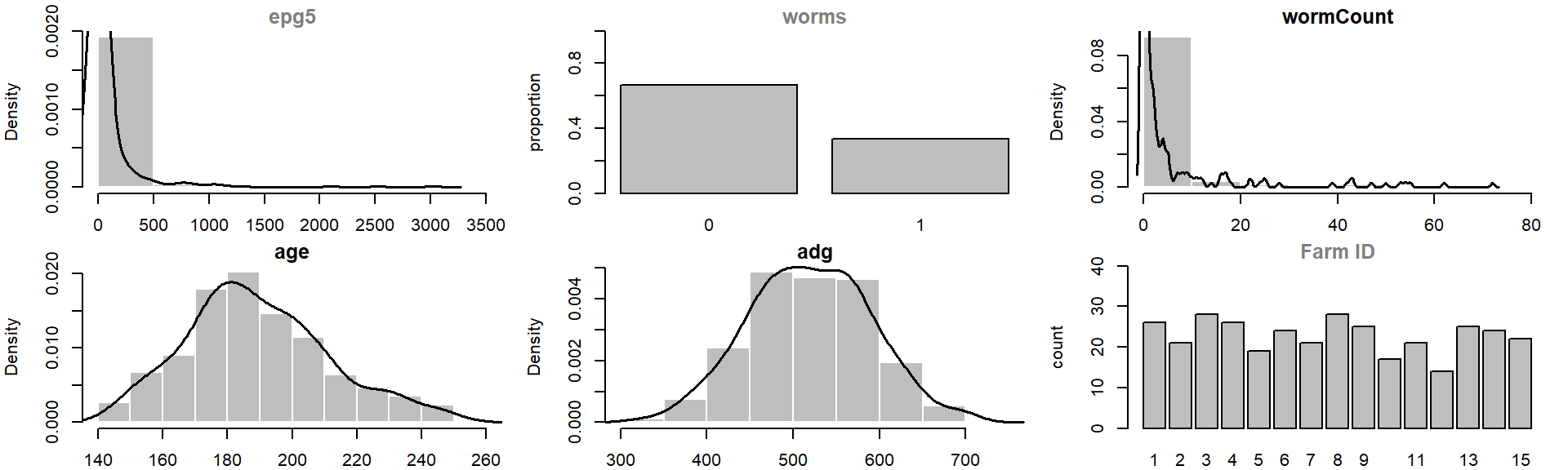For this exercise we will drop some variables, namely pneum, eggs, wormCount and farm:

drop <- which(colnames(dt)%in% c("pneum", "epg5", "worms", "farm"))
abndata <- dt[, -drop]

### 0) Set all binary variables to FACTOR (and check that dataset is complete)

A requirement of abn (Kratzer, Pittavino, Lewis, & Furrer, 2017; Lewis & Ward, 2013) is that all binary variables are coerced into factors.

str(abndata)
Classes 'tbl_df', 'tbl' and 'data.frame':   341 obs. of  8 variables:
$AR : num 1 1 0 1 1 1 1 1 1 1 ...$ pneumS   : num  0 0 0 0 1 0 0 0 0 0 ...
$female : num 0 0 1 1 0 0 1 1 1 0 ...$ livdam   : num  1 1 1 1 0 0 0 1 1 0 ...
$eggs : num 0 0 0 0 0 0 0 0 0 0 ...$ wormCount: num  0 0 0 0 0 0 0 0 0 0 ...
$age : num 196 175 176 207 222 212 247 181 191 181 ...$ adg      : num  453 501 545 441 482 423 407 580 427 586 ...
abndata[,1:5] <- as.data.frame(lapply(abndata[,1:5], factor))
sum(complete.cases(abndata))
 341

### 1) Create network matrix

Let’s start by creating a network matrix, where the result of the model search (i.e. optimal DAG) will be stored. The size of the matrix will be equal to the variables to be included in the model, i.e. the number of columns of the dataframe containing our data to be analysed (abndata). The network matrix needs also to have named rows and columns, with the same names as in the abndata dataframe.

dag <- matrix(0, ncol(abndata), ncol(abndata))
colnames(dag) <- rownames(dag) <- names(abndata)

### 2) Setup the distribution list for each variable

Each variable in the model needs to be associated to a distribution (currently available: binomial, Gaussian, Poisson) according to the type of data. In this example most of the variables are binary and therefore associated to the binomial distribution. Variables age and adg are continuous and fairly normally distributed, so they will be associated to the Gaussian distribution. Finally, variable wormCount is a count and can be approximated by a Poisson distribution.

dist <- list(AR = "binomial", pneumS = "binomial", female="binomial",
livdam= "binomial", eggs = "binomial",wormCount = "poisson",
age= "gaussian", adg = "gaussian")

### 3) Create retain and banned matrixes (empty)

Prior knowledge about data structure, that could guide the search for the optimal model, can be included by forcing or banning some specific arcs from being considered in the final DAG. This is done by providing a retain matrix and/or a ban matrix. We will start by creating two empty matrices with the same size as our data (8) and named rows and columns.

retain <- matrix(0, ncol(abndata), ncol(abndata))
colnames(retain) <- rownames(retain) <- names(abndata)

banned <- matrix(0, ncol(abndata), ncol(abndata))
colnames(banned) <- rownames(banned) <- names(abndata)

### 4) Ban some arcs (optional)

The information encoded in the ban matrix is subjectively chosen to reflect our belief about data structure. In this example, it is reasonable to assume that none of the variables in the model is going to affect the gender of the animal (which is an inborn trait). To encode this information we will ban all the arcs going to female, by setting their value to 1 (banned) as opposite to the default value 0 (non banned).

How does it work?

Rows are children, columns parents:
. b1 b2 b3 b4
b1 0 1 0 0
b2 0 0 0 0
b3 1 0 0 0
b4 0 0 0 0

So ban[1, 2] <- 1 means do not allow the arc from b2 (second column) to b1 (first row) and ban[3, 1] <- 1 means do not allow the arc from b1 (first column) to b3 (third row).

Now, we want to ban the arcs going from any variable (= all columns except the third) to female (= third row):

banned[3,-3] <- 1

Have a look if you want:

banned
          AR pneumS female livdam eggs wormCount age adg
AR         0      0      0      0    0         0   0   0
pneumS     0      0      0      0    0         0   0   0
female     1      1      0      1    1         1   1   1
livdam     0      0      0      0    0         0   0   0
eggs       0      0      0      0    0         0   0   0
wormCount  0      0      0      0    0         0   0   0
age        0      0      0      0    0         0   0   0
adg        0      0      0      0    0         0   0   0

### 6) Run the exact search across incremental parent limits

Repeat step 5 for incremental parent limit (e.g. 1 to nr.var-1). The optimal DAG is the one where the network score does not improve (i.e. becomes bigger) any longer by allowing more parents.

datadir <- tempdir()

for (i in 1:7) {
max.par <- i

mycache <- buildscorecache(data.df = as.data.frame(abndata), data.dists = dist,
dag.banned = banned, dag.retained = retain,
max.parents = max.par)

mydag <- mostprobable(score.cache = mycache)

fabn <- fitabn(dag.m = mydag, data.df = as.data.frame(abndata), data.dists = dist)

cat(paste("network score for", i, "parents =", fabn$mlik, "\n\n")) save(mycache, mydag, fabn, file = paste(datadir,"mp_", max.par,".RData", sep="")) } Step1. completed max alpha_i(S) for all i and S Total sets g(S) to be evaluated over: 256 network score for 1 parents = -2806.49909464438 Step1. completed max alpha_i(S) for all i and S Total sets g(S) to be evaluated over: 256 network score for 2 parents = -2714.69171704675 Step1. completed max alpha_i(S) for all i and S Total sets g(S) to be evaluated over: 256 network score for 3 parents = -2709.3707884251 Step1. completed max alpha_i(S) for all i and S Total sets g(S) to be evaluated over: 256 network score for 4 parents = -2709.25275104164 Step1. completed max alpha_i(S) for all i and S Total sets g(S) to be evaluated over: 256 network score for 5 parents = -2709.25275104164 Step1. completed max alpha_i(S) for all i and S Total sets g(S) to be evaluated over: 256 network score for 6 parents = -2709.25275104164 Step1. completed max alpha_i(S) for all i and S Total sets g(S) to be evaluated over: 256 network score for 7 parents = -2709.25275104164  ### 7) Retrieve maximum marginal likelihood to compare models # get network score for all parent limits # --------------------------------------- mp.mlik <- c() for (i in 1:max.par) { load(paste(datadir,"mp_", i,".RData", sep="")) mp.mlik <- c(mp.mlik, fabn$mlik)
}

# check how it looks
# ------------------
plot(1:max.par, mp.mlik, xlab = "Parent limit", ylab = "Log marginal likelihood",
type = "b", col="red", ylim=range(mp.mlik))
abline(v=which(mp.mlik==max(mp.mlik)), col="grey", lty=2)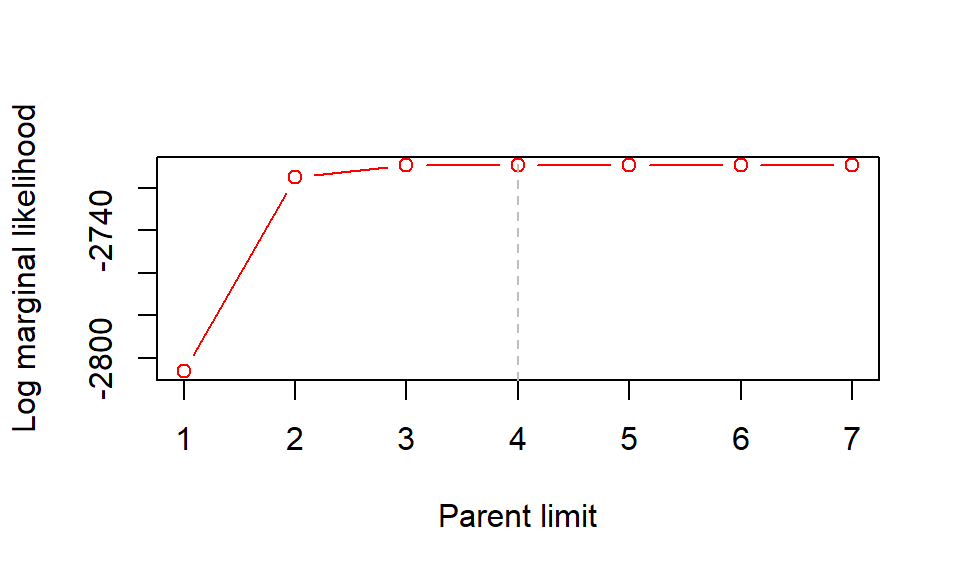After max.par=4 the maximum log marginal likelihood is constant:

 -2806.499 -2714.692 -2709.371 -2709.253 -2709.253 -2709.253 -2709.253

### 8) Retrieve best fitting model and visualize DAG

max.par<- which(mp.mlik==max(mp.mlik))
load(paste(datadir,"mp_", max.par,".RData", sep=""))

tographviz(dag.m = mydag, data.df = abndata, data.dists = dist,
outfile = paste("DAG_", which(mp.mlik==max(mp.mlik)),
"p.dot", sep=""))

The function tographviz()creates a .dot file which can be further read with the external software GraphViz (Ellson, Gansner, Koutsofios, North, & Woodhull, 2001). Here is what the best fitting DAG looks like: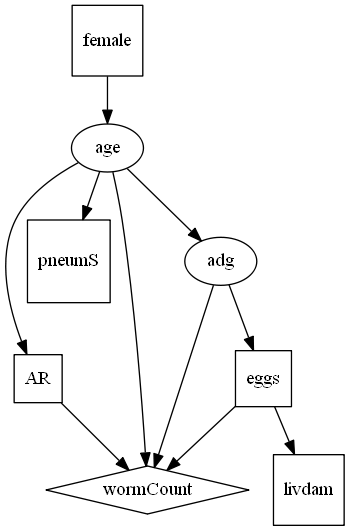Before going ahead interpreting the results, we need one more step. So far, we have identified a DAG which has the maximum possible goodness of fit according to the log marginal likelihood. This is the standard goodness of fit metric in Bayesian modelling and includes an implicit penalty for model complexity. However, the log marginal likelihood is also known to be prone to overfitting (especially with smaller data sets), meaning that it may identify more parameters than can be actually justified by the data. Therefore, it is advisable to check and address potential overfitting before drawing any conclusion based on the model results.

A well established approach for addressing overfitting is to use parametric bootstrapping. Basically, the model chosen from the exact search will be used to generate many bootstrap datasets (e.g. 5000) of equal size to the original dataset (n=341 in our case). Each bootstrap dataset will be then treated as if it were the original data, and a globally optimal DAG will be identified exactly as described before (i.e. exact search). We will therefore get as many DAGs as the number of simulated datasets (eg. 5000). To address overfitting, any arcs in the DAG from the original data which will not be recovered in > 50% of the bootstrap DAGs will be deemed to have insufficient statistical support to be considered robust.

### 9) Parametric bootstrapping

This step will be done with the aid of a software for Bayesian statistical analysis using Markov Chain Monte Carlo (MCMC) simulations (we will use JAGS, but any other is fine). We will use the parameters estimated from our model to build a BUG model to simulate data. In other words, we will do the reverse process: instead of using data to estimate parameters, we will use parameters to estimate data.

#### 9.1) Extract parameters from best fitting model and save them for MCMC simulations

The parameters at each node are estimated as posterior probability density distributions. These marginal densities are the ones where JAGS needs to sample from in order to simulate data. In order to use these distributions with JAGS, the densities need to be approximated by a discrete distribution over a fine and equally spaced grid. Here is a mock example for a hypothetical parameter $$\beta$$: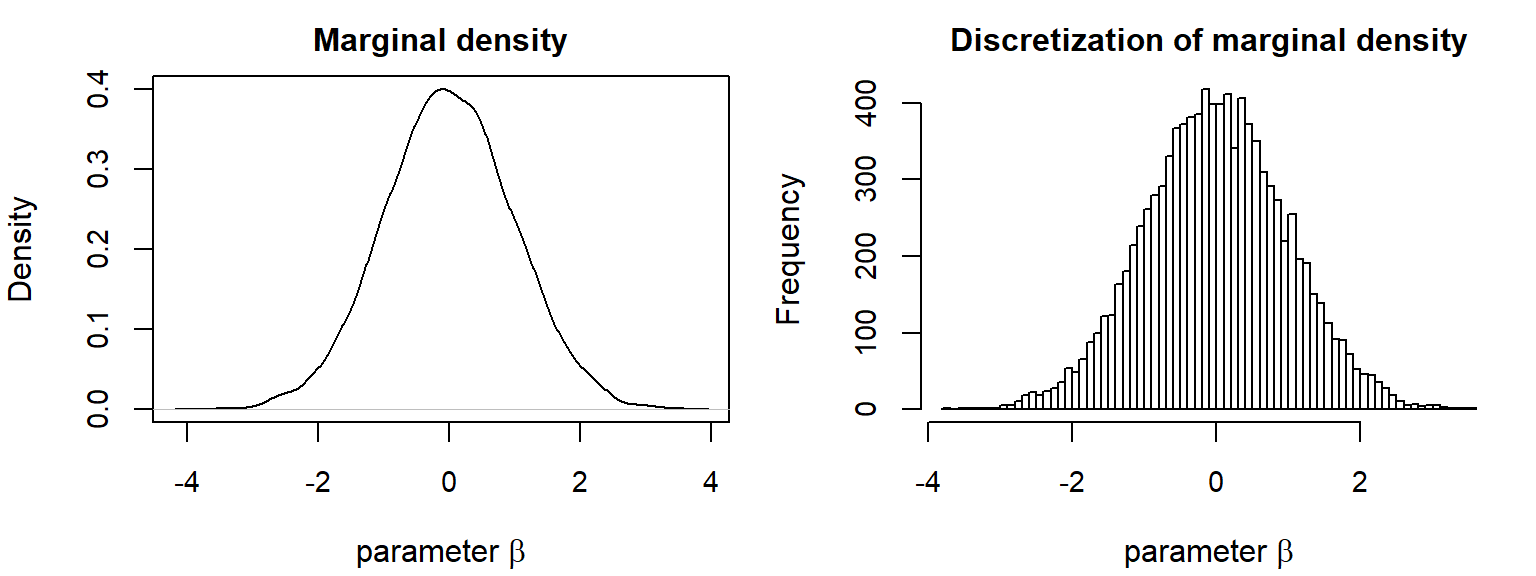# Fit marginal densities over a fixed grid --> n.grid=1000
# --------------------------------------------------------
marg.f <- fitabn(dag.m = mydag, data.df = as.data.frame(abndata),
data.dists = dist, compute.fixed=TRUE, n.grid=1000)

# Extract values
# --------------
m <- marg.f$marginals[] for(i in 2: length(marg.f$marginals))
{ m <- c(m, marg.f$marginals[[i]])} # Bind all the marginals for the same node into a matrix # ------------------------------------------------------ AR.p <- cbind( m[[ "AR|(Intercept)"]], m[[ "AR|age"]]) pneumS.p <- cbind( m[[ "pneumS|(Intercept)"]], m[[ "pneumS|age"]]) female.p <- cbind( m[[ "female|(Intercept)"]]) livdam.p <- cbind( m[[ "livdam|(Intercept)"]], m[[ "livdam|eggs"]]) eggs.p <- cbind( m[[ "eggs|(Intercept)"]], m[[ "eggs|adg"]]) wormCount.p <- cbind( m[[ "wormCount|(Intercept)"]], m[[ "wormCount|AR"]], m[[ "wormCount|eggs"]], m[[ "wormCount|age"]], m[[ "wormCount|adg"]]) age.p <- cbind( m[[ "age|(Intercept)"]], m[[ "age|female"]]) prec.age.p <- cbind( m[[ "age|precision" ]]) adg.p <- cbind( m[[ "adg|(Intercept)"]], m[[ "adg|age"]]) prec.adg.p <- cbind( m[[ "adg|precision" ]]) # Save it to a file named PostParams to be read by JAGS # ----------------------------------------------------- dump(c("AR.p", "pneumS.p", "female.p", "livdam.p", "eggs.p", "wormCount.p", "age.p", "prec.age.p", "adg.p", "prec.adg.p"), file="PostParams.R") #### 9.2) Write the BUG model In order to simulate the variables of our dataset we need to provide a model for each of them, using the aforementioned parameters estimates. For instance, the binomial node AR in our DAG has one incoming arc coming from the node age. In a regression notation this would be translated into: logit(AR) = $$\alpha$$ + $$\beta$$ x age + $$\epsilon$$ where $$\alpha$$ is the intercept and $$\beta$$ the regression coefficient for variable age and $$\epsilon$$ is the error term modelled by a binomial distribution. Given that we will simulate the data in a Bayesian framework, AR will be modelled as a probability distribution. Therefore it will look like: AR ~ dbern(probAR); logit(probAR)<- alpha + beta*age; Then, the values of alpha and beta will be sampled (dcat) from our discrete distribution of parameters: alpha.prob ~ dcat(AR.p[ ,2]); –> sample from the vector of density values f(x) (second column in matrix) alpha ~ AR.p[alpha.prob,1]; –> corresponding x value for the sampled density (first column in matrix) beta.prob ~ dcat(AR.p[ ,4]); beta ~ AR.p[beta.prob,1]; The BUG file (model8vPois.bug) can be retrieved from the file directory. #### 9.3) Run JAGS and inspect the result of a simulated dataset library(rjags) # set inits # --------- init <- list(".RNG.name"="base::Mersenne-Twister", ".RNG.seed"=42) # load data # --------- source("PostParams.R") # run model once # -------------- jj <- jags.model(file = "model8vPois.bug", data = list( 'AR.p'=AR.p , 'pneumS.p'=pneumS.p , 'female.p'=female.p, 'livdam.p'=livdam.p , 'eggs.p'=eggs.p , 'wormCount.p'=wormCount.p , 'age.p'=age.p ,'prec.age.p'=prec.age.p, 'adg.p'=adg.p , 'prec.adg.p'=prec.adg.p), inits = init, n.chains = 1, n.adapt = 5000) Compiling model graph Resolving undeclared variables Allocating nodes Graph information: Observed stochastic nodes: 0 Unobserved stochastic nodes: 28 Total graph size: 40091 Initializing model # run more iterations # ------------------- update(jj, 100000) # set number of observation we want to extract for a dataset # ---------------------------------------------------------- n.obs=341 # sample data (same size as original: 341) with a sampling lag (20) to reduce avoid autocorrelation # ------------------------------------------------------------------------------------------------- samp <- coda.samples(jj, c("AR", "pneumS", "female", "livdam", "eggs", "wormCount", "age", "prec.age", "adg", "prec.adg"), n.iter= n.obs*20 , thin =20) Now compare the simulated data with the original data. Observe that Gaussian nodes are by default centred when doing abn search, meaning that the simulated data for those nodes will be centred as well.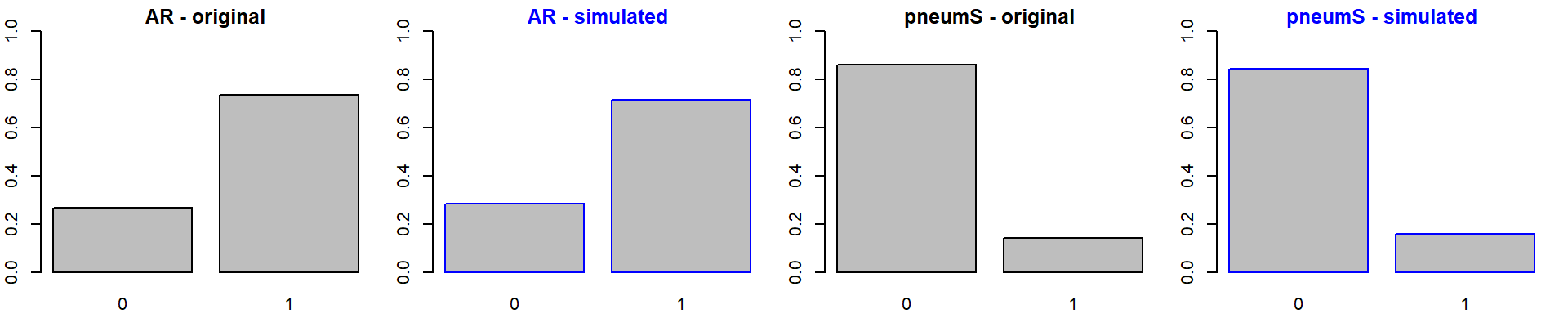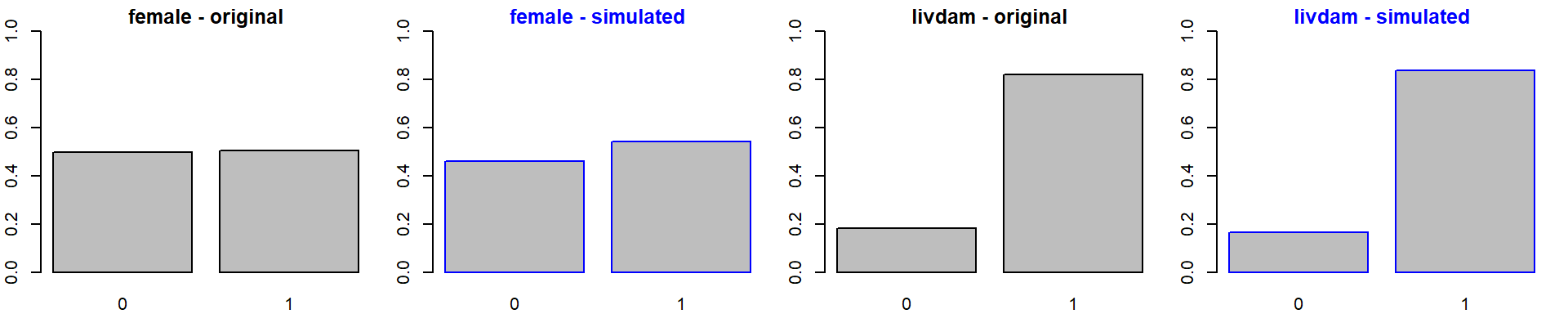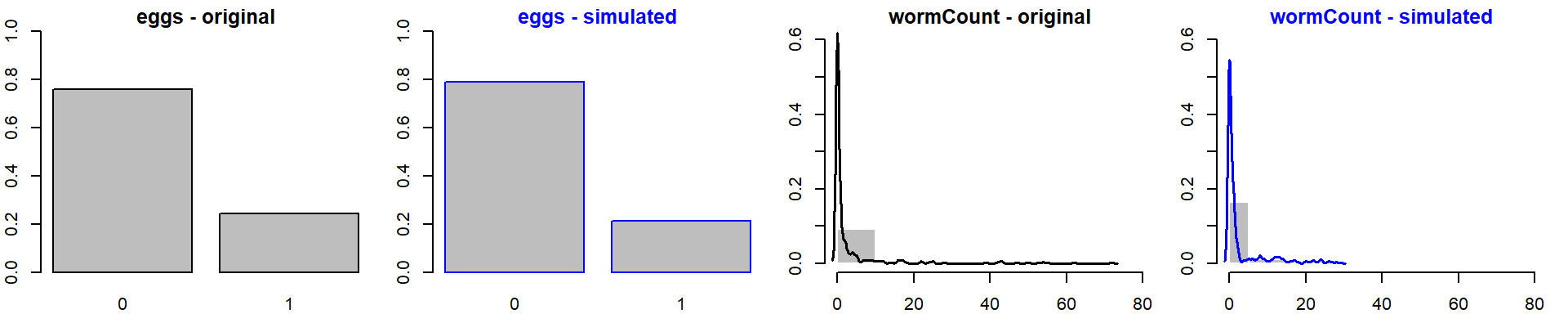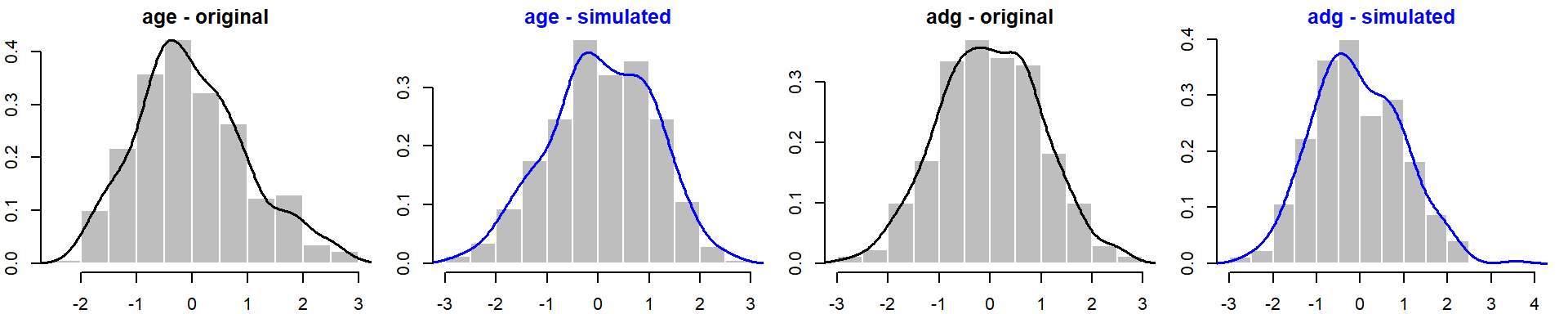Simulated data looks fairly OK (perhaps wormCount is sub-optimal as the simulated data miss to represent the long right tail) so we can proceed with the bootstrapping. #### 9.4) Iterate dataset simulation + exact search over and over What we will do is to create a loop to 1) simulate data, 2) do exact search on such data, and 3) store the best fitting DAG over and over for many times (e.g. 5000 iterations). Bootstrap data need to be saved in a folder to be further inspected. vars <- colnames(abndata) # load data for jags source("PostParams.R") # select nr. bootstrap samples to run # ----------------------------------- set.seed(123) # get 5000 random numbers to set different initial values # ------------------------------------------------------- n <- sample(1:100000, 5000) # specify max number of parents based on previous search # ------------------------------------------------------ max.par <- 4 # Simulate data and run ABN on such dataset # ----------------------------------------- for (i in 1:length(n)) { print(paste("/n Running simulation", i)) # pick initials init <- list(".RNG.name"="base::Mersenne-Twister", ".RNG.seed"=n[i]) # run model jj <- jags.model(file = "model8vPois.bug", data = list( 'AR.p'=AR.p , 'pneumS.p'=pneumS.p , 'female.p'=female.p, 'livdam.p'=livdam.p , 'eggs.p'=eggs.p , 'wormCount.p'=wormCount.p , 'age.p'=age.p ,'prec.age.p'=prec.age.p, 'adg.p'=adg.p , 'prec.adg.p'=prec.adg.p), inits = init, n.chains = 1, n.adapt = 5000) # run more iterations update(jj, 100000) # sample data (same size as original: 341) with a sampling lag (20) to reduce avoid autocorrelation samp <- coda.samples(jj, c("AR", "pneumS", "female", "livdam", "eggs", "wormCount", "age", "prec.age", "adg", "prec.adg"), n.iter= 6820 , thin =20) # build dataframe in the same shape as the original one dt.boot <- as.data.frame(as.matrix(samp)) # pay attention at order names dt.boot<- dt.boot[, vars] # now coerce to factors if need be and set levels - NOTES setting levels works as # "0" "1" is in the same order as "absent" "present" from original data abndata <- as.data.frame(abndata) for(j in 1:length(vars)) { if(is.factor(abndata[,j])) { dt.boot[,j]<-as.factor(dt.boot[,j]); levels(dt.boot[,j])<-levels(abndata[,j]); } } # Build a cache of all local computations # --------------------------------------- mycache <- buildscorecache(data.df = dt.boot, data.dists = dist, dag.banned = banned, dag.retained = retain, max.parents = max.par) # Run the EXACT SEARCH # -------------------- mp.dag <- mostprobable(score.cache = mycache) fabn <- fitabn(dag.m = mp.dag, data.df = dt.boot, data.dists = dist) # Save the results obtained # ------------------------- save(mycache, mp.dag, fabn, dt.boot, file = sprintf('BootData/dt.boot.%04d.RData',i)) } #### 9.5) Find the final pruned DAG First we need to load all the bootstrap DAGs. # set nr bootstrap samples # ------------------------ n <- 5000 # load dags and boostrap data # ------------------------------ dags <- list() boot <- list() for (i in 1:n) { load(file = sprintf('BootData/dt.boot.%04d.RData',i)) dags[[i]] <- mp.dag boot[[i]] <- dt.boot rm(mp.dag, dt.boot, fabn, mycache) }  Then we will check what was the most frequent number of arcs: # count total number of arcs in each dag # -------------------------------------- arcs <- sapply(dags, sum) barplot(table(arcs))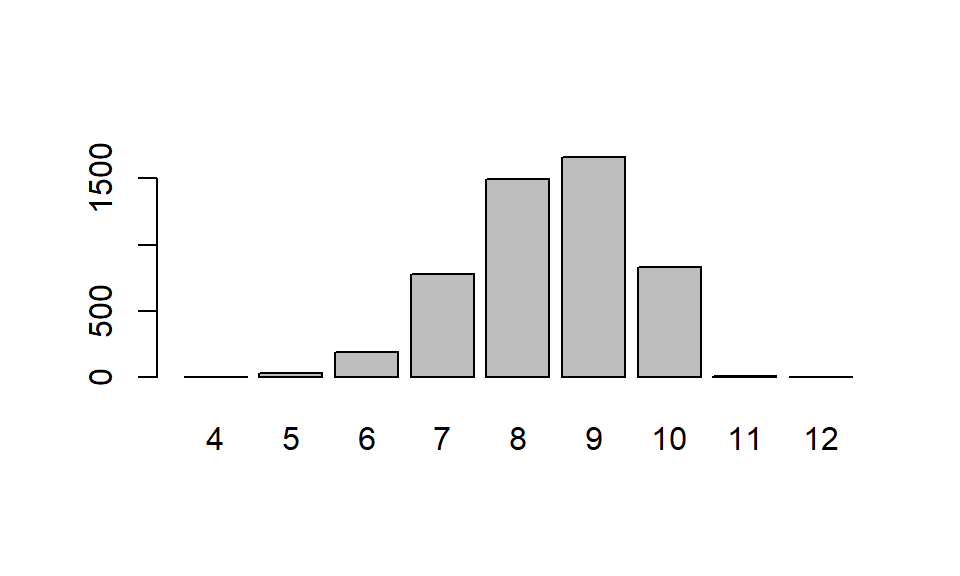For comparison, in the original DAG there were 10 arcs, so it seems that there might have been some over-fitting. Finally, we will count how many times each arc appears in the bootstrap data. The final pruned DAG will include only arcs present in at least 50% of bootstrap samples (e.g. 2500/5000 in our case). # Count how many times each arc appear in the bootstrap data # ---------------------------------------------------------- # function Reduce sums ("+") each element of each maxtrix in the list and store # results in a new matrix of same size alldag <- Reduce("+", dags) # express it in percentage perdag <- alldag/length(dags) # Keep only arcs that appears in at least 50% of samples # ------------------------------------------------------ trim.dag <- (alldag >=(n*0.5))*1 # sum(trim.dag) # Send final pruned DAG to Graphvis for visualization # ----------------------------------------------------- tographviz(dag.m = trim.dag, data.df = abndata, data.dists = dist, outfile = "TrimDAG.dot") This is the percentage of arcs retrieved within the bootstrap sample:  AR pneumS female livdam eggs wormCount age adg AR 0 0 0 0 0 0 76 5 pneumS 0 0 1 0 0 0 39 8 female 0 0 0 0 0 0 0 0 livdam 0 1 0 0 54 2 0 0 eggs 0 0 0 24 0 0 8 75 wormCount 71 1 0 0 100 0 100 61 age 18 17 56 0 4 0 0 25 adg 1 2 10 0 6 0 75 0 This is how the final pruned DAG looks like: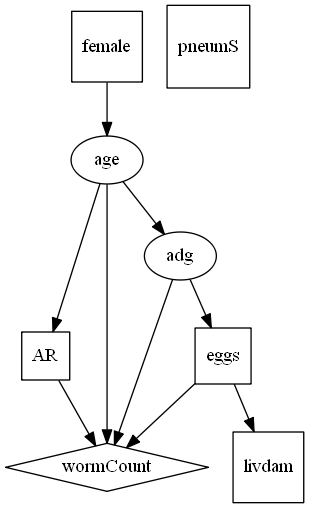Now that we have a robust model (encoded by the pruned DAG), we can extract the parameters to (a) appreciate the magnitude (and precision) of the the associations and (b) further refine the DAG including whether the associations are positive or negative. ### 10) Extract marginal posterior density for each parameter The marginal posterior densities (marginals) represent the density distribution of the parameters at each arc. marg.f <- fitabn(dag.m = trim.dag, data.df = as.data.frame(abndata), data.dists = dist, compute.fixed=TRUE, n.grid=1000) #### 10.1) Visually inspect the marginal posterior distributions of the parameters par(mfrow=c(1,4), mar=c(2,2,1.5,1)) for(i in 1:length(marg.f$marginals)){

# get the marginal for current node, which is a matrix [x, f(x)]
cur.node <- marg.f$marginals[i] nom1 <- names(marg.f$marginals)[i]

# pick the first value (for models wothout random effects)
cur.node <- cur.node[]
for(j in 1:length(cur.node) ) {
nom2<-names(cur.node)[j]
cur.param <- cur.node[[j]]
plot(cur.param,type="l",main=paste(nom1, ":", nom2), cex=0.7)
}
}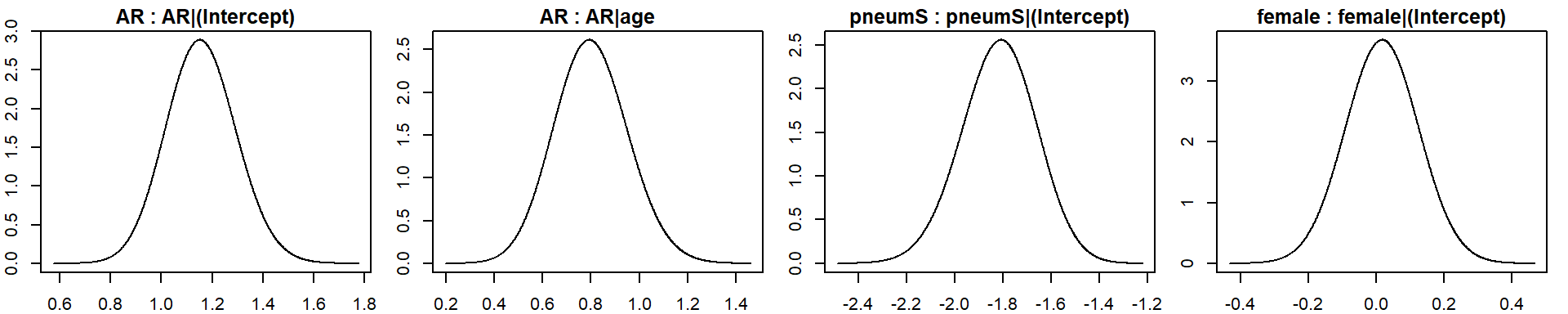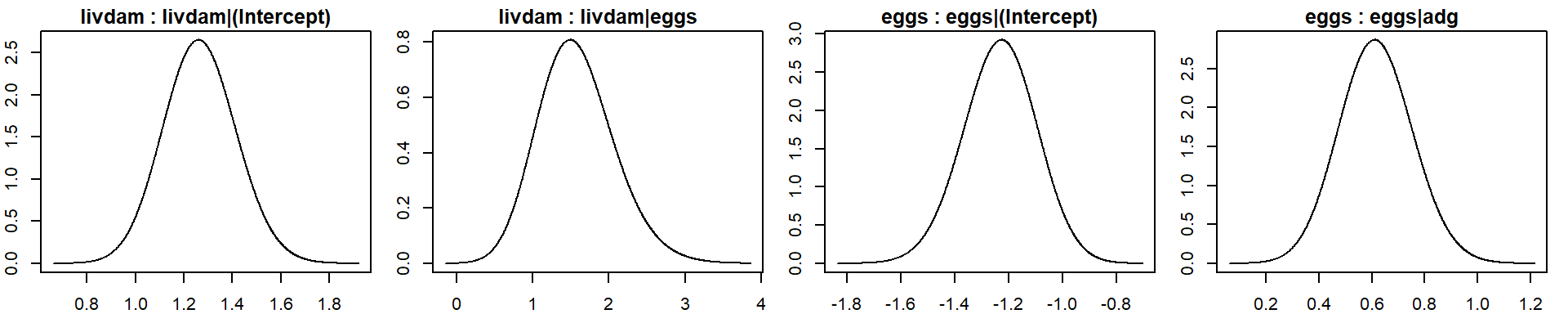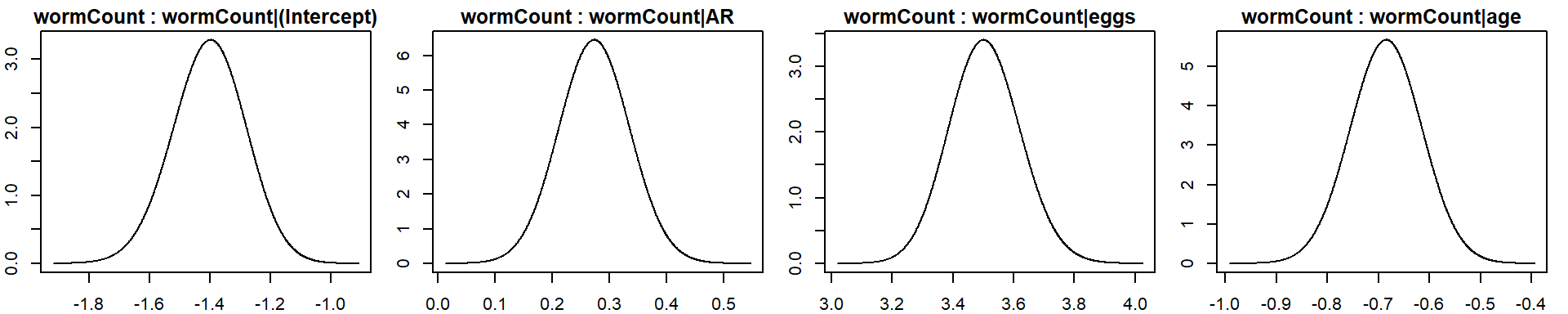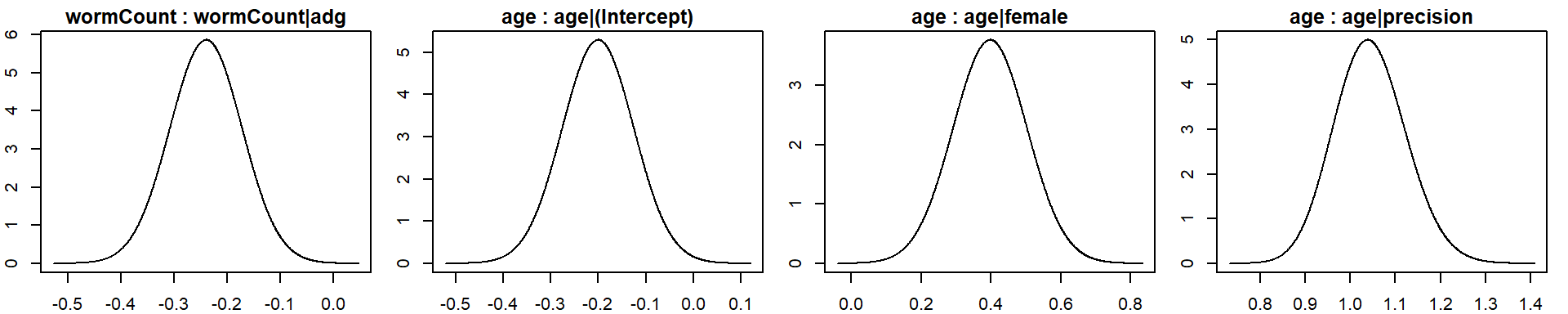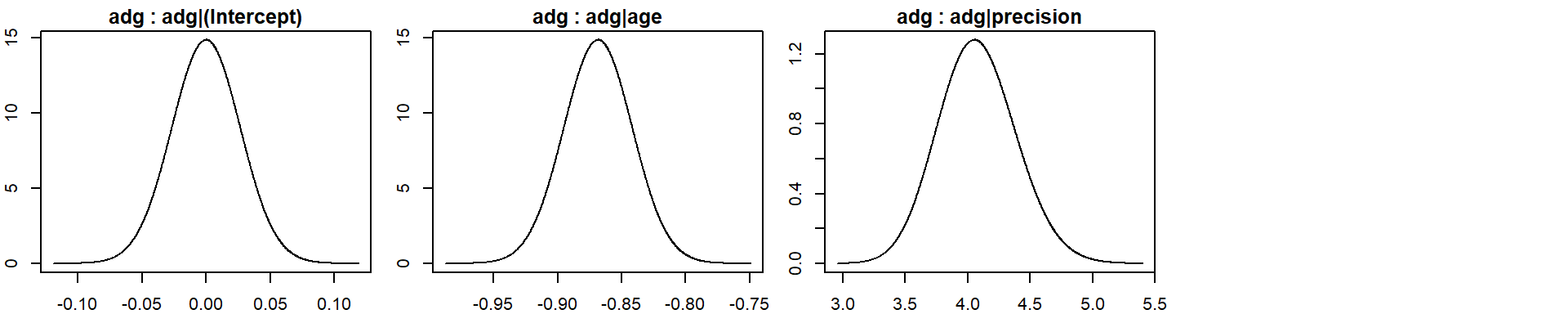#### 10.2) Calculate the area under the density curve

# extract marginals
marg.dens <- marg.f$marginals[] for (i in 2:length(marg.f$marginals)) {
marg.dens <- c(marg.dens, marg.f$marginals[[i]]) } # calculate AUC --> should be ~1 auc <- rep(NA, length(marg.dens)) names(auc) <- names(marg.dens) for(i in 1:length(marg.dens)) { tmp <- spline(marg.dens[[i]]) auc[i] <- sum(diff(tmp$x[-length(tmp$x)])*tmp$y[-1])
}

cbind(auc)
                            auc
AR|(Intercept)        0.9999997
AR|age                0.9999994
pneumS|(Intercept)    0.9999990
female|(Intercept)    0.9999994
livdam|(Intercept)    0.9999995
livdam|eggs           0.9999984
eggs|(Intercept)      0.9999993
eggs|adg              0.9999993
wormCount|(Intercept) 0.9999993
wormCount|AR          0.9999996
wormCount|eggs        0.9999997
wormCount|age         0.9999996
wormCount|adg         0.9999996
age|(Intercept)       0.9999995
age|female            0.9999992
age|precision         0.9999996
adg|(Intercept)       0.9999998
adg|age               0.9999998
adg|precision         0.9999985
par(las=2, mar=c(8.1,4.1,4.1,2.1))
barplot(auc, ylab="Area under Density", ylim=c(0,1.2))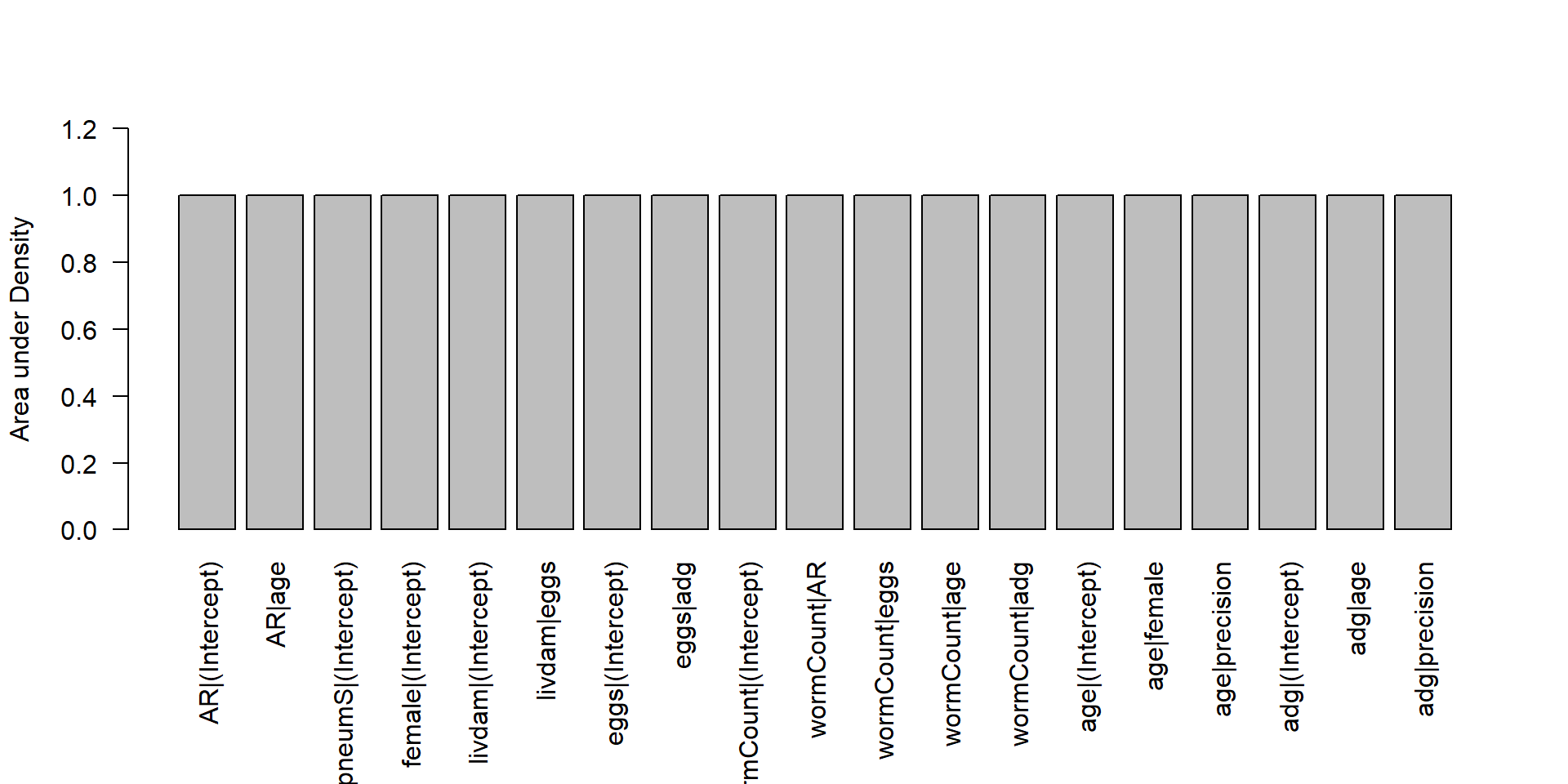Both the shape of the parameter density distributions and their AUC looks satisfactory, so we can proceed getting the estimates.

#### 10.3) Get the table of quantiles for the marginals

mat <- matrix(rep(NA, length(marg.dens)*3), ncol=3)
rownames(mat) <- names(marg.dens)
colnames(mat) <- c("2.5%", "50%", "97.5%")
ignore.me <- union(grep("\\(Int", names(marg.dens)), grep("prec", names(marg.dens))) # take away background k and precision
comment <- rep("", length(marg.dens))
for (i in 1:length(marg.dens)) {
tmp <- marg.dens[[i]]
tmp2 <- cumsum(tmp[,2])/sum(tmp[,2])
mat[i, ] <-c(tmp[which(tmp2>0.025)-1,1],## -1 is so use value on the left of the 2.5%
tmp[which(tmp2>0.5),1],
tmp[which(tmp2>0.975),1])
vec <- mat[i,]

if( !(i%in%ignore.me) &&  (vec<0 && vec>0)){comment[i]<-"not sig. at 5%"}

## truncate for printing
mat[i,]<-as.numeric(formatC(mat[i,],digits=3,format="f"))
}

cbind(mat)
                        2.5%    50%  97.5%
AR|(Intercept)         0.891  1.155  1.435
AR|age                 0.509  0.798  1.110
pneumS|(Intercept)    -2.134 -1.814 -1.519
female|(Intercept)    -0.196  0.018  0.230
livdam|(Intercept)     0.976  1.265  1.567
livdam|eggs            0.663  1.547  2.656
eggs|(Intercept)      -1.508 -1.232 -0.971
eggs|adg               0.346  0.614  0.895
wormCount|(Intercept) -1.646 -1.400 -1.170
wormCount|AR           0.152  0.273  0.395
wormCount|eggs         3.280  3.504  3.741
wormCount|age         -0.824 -0.685 -0.548
wormCount|adg         -0.374 -0.240 -0.107
age|(Intercept)       -0.349 -0.200 -0.052
age|female             0.188  0.397  0.606
age|precision          0.893  1.042  1.207
adg|(Intercept)       -0.053  0.000  0.053
adg|age               -0.921 -0.868 -0.816
adg|precision          3.485  4.070  4.712

As said, the marginals represent estimates of the parameters at each node (i.e. the arcs in the DAG). Being the variables of different nature, they have different meaning. Marginals represent correlation coefficients for Gaussian nodes (when the default centering of variables is kept), log rate ratios for Poisson nodes, and log odds ratios for binomial nodes. Therefore, the second and the latter need to be exponentiated, to get the odds ratios or rate ratios respectively.

### 12) Present final results

As a final step we will refine the DAG by (a) changing the style of the arrows according to the type of association (i.e. solid arrows for positive association and dashed arrows for negative association), and (b) changing the thickness of the arrows according to their link strength. These steps are done in Graphviz, using the DOT language. Details can be found at https://www.graphviz.org/doc/info/attrs.html.